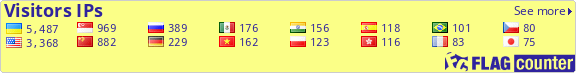## Number 1018, 2012

T.I. Smortsova, Mapping of the linear systems of the second order with two-dimensional control, P. 4-20.

This paper deals with linear control systems with two-dimensional control. The main goal of the paper is to find the exact analytic solution of the time-optimal control problem for an arbitrary linear control system with constant coefficients using the analytic solution of this problem for the simplest system. For this aim, we construct a certain nonsmooth mapping between the 0-controllability sets of the given systems. In other words, by means of this mapping, we investigate the equivalence of the systems with the same qualitative behavior in a neighborhood of the stationary point.

Keywords: Linear control system, two-dimensional control.

V.D.Gordevskyy, Yu.A. Sysoyeva, Rapidly relaxing solutions of the Boltzmann equation for a gas of hard spheres, P. 21-29.

The new approximate solution for the nonlinear kinetic Boltzmann equation in the case of the model of hard spheres is found. It ensures the infinitesimality of the uniform-integral weighted discrepancy between the sides of the equation and very fast tends to zero with increasing of the time.

Keywords: Hard spheres, Boltzmann equation, discrepancy.

A.S. Sokhin, Identification of distributed parameter systems, described by the equation of neutron transport with anisotropic time-dependent collision integral, P. 30-40.

In the paper we study the problem of identification of a nonstationary many-dimensional linear Boltzmann equation with a nonsingular generator of neutrons. We identify an equation by the given initial flows of neutrons and the observed final flows of neutrons. We ascertain the one-to-one correspondence between density of the generator of neutrons and the observation which associates inicial increment of neutrons to the final flow of neutrons.

Keywords: Identication, equation of transport.

V. N. Sirovatsky, Functional models of commutative systems of operators close to the unitary, P. 41-61.

For commutative system of the linear limited operators \$ T_1, T_2 \$ which operate in Hilbert space H, and not one of operators \$ T_1, T_2 \$ is compression, the functional model is constructed. This model is build in space of Louis De Branges for a circle.

Keywords: Operator, functional model.

E.V. Oliynyk, The solution of non-linear equations of a special kind, P. 62-75.

The solutions of the system of non-linear equations, basis for the construction of triangular models used for the commutative systems of non-self-conjugate operators were studied and described in this work.

Keywords: Triangular, commutative systems of linear nonselfadjoint operators.

B.V. Uspensky, K.V. Avramov, Nonlinear normal modes of forced oscillations of piecewise linear systems, P. 76-83.

A method of analysis of nonlinear normal modes for forced oscillations in piecewise linear systems is proposed. The method is based at Rauscher technique that turns non-authonomous dynamical system to an iterative process. Each step of this process resolves an authonomous dynamical system.

Keywords: Nonlinear normal modes, piecewise linear systems, forced oscillations.

Back to the Journal's main page.
;   Different visitors (IPs) since May 2, 2015: